## Chapter Goals

• To learn about the method of recursion
• To understand the relationship between recursion and iteration
• To analyze problems that are much easier to solve by recursion than by iteration
• To learn to "think recursively"
• To be able to use recursive helper functions
• To understand when the use of recursion affects the efficiency of an algorithm

## Triangle Numbers

• In this example, we will look at triangle shapes such as the one shown here:
`[][][][][][]`
• The n-th triangle number is the area of a triangle of width n.
• From above, the third triangle number is 6.
• Here is the outline of the class that we will develop:
`class Triangle {public:   Triangle(int w);   int get_area() const;private:   int width;};Triangle::Triangle(int w){ width = w; }`
• If the width of the triangle is 1, then the triangle has an area of 1.
`[]int Triangle::get_area(){ if (width = = 1) return 1;   . . .}`
• To deal with the general case, consider this picture.
`[][][][][][][][][][]`
• We think of area of the larger triangle as
`smaller_area + width`
• To find the smaller area, we make a smaller triangle!
`int Triangle::get_area(){  if (width == 1) return 1;   Triangle smaller_triangle(width - 1);   int smaller_area = smaller_triangle.get_area();   return smaller_area + width;}`
• Here's how the area is computed for a triangle of width 4.
• The get_area function makes a smaller triangle of width 3.
• It calls get_area on that triangle.
• That function makes a smaller triangle of width 2.
• It calls get_area on that triangle.
• That function make a smaller triangle of width 1.
• It calls get_area on that triangle.
• That function returns 1.
• The function returns smaller_area + width = 1 + 2 = 3.
• The function returns smaller_area + width = 3 + 3 = 6.
• The function returns smaller_area + width = 6 + 4 = 10.
• The technique of expressing a solution to a problem in terms of solution to a smaller version of the same problem is called recursion.
• There are two key requirements to make that recursion is successful:
• Every recursive call must simplify the computation in some way.
• It must be a special case to handle the simplest computations directly.
• Here, get_area calls itself again with smaller and smaller width values, eventually reaching a width of 1.
• Recursion is not really necessary to solve this problem:
• A simple loop:
`double area = 0;for (int i = 1; i <= width; i++) area = area + 1;`
• A formula:
`width * (width + 1) / 2`

## Permutations

• We will design a function that lists all permutations of a string.
• A permutation is simply a rearrangement of the letters:
`"eat""eta""aet""ate""tea""tae"`
• If a string has n letters, then the number of permutations is given by the factorial function:
`n! = 1 x 2 x 3 x . . . x n`
• To a compute the value of a n! you could use a loop (see chapter 7), but there is also a recursive solution:
`n! = (n - 1)! x n `
• It is customary to define
`1! = 10! = 1`
• You can therefore implement a recursive factorial function (which counts how many permutations there are) as follows:
`int factorial(int n){  if (n == 0) return 1;   int smaller_factorial = factorial(n - 1);   int result = smaller_factorial * n;   return result;}`
• We will develop a function that generates all permutations of a word.
`vector<string> generate_permutations(string word);`
• The following code displays all permutations of the string "eat":
`vector<string> v = generate_permutations("eat");for(int i = 0; i < v.size(); i++)   cout << v[i] << "\n";`
• To generate the permutations recursively, generate all permutations that state with the letter 'e', the those that start with 'a', then those that start with 't'.
• Use the recursion to generate all the permutations of the shorter (two-letter) strings.
• "at" "ta"
• "et" "te"
• "ae" "ea"
• Add the first letters to find the permutations.
• "eat" "eta"
• "aet" "ate"
• "tae" "tea"
• To carry out the ideas in the previous example, we implement a loop that creates a shorter word that omits the current position.
`vector<string> generate_permutations(string word){  vector<string> result;   ...   for (int i = 0; i < word.length(); i++)   {     string shorter_word = word.substr(0, i)         + word.substr(i + 1, word.length() - i - 1);      ...   }   return result;}`
• The next step is to compute the permutations of the shorter word.
`vector<string> shorter_permutations   = generate_permutations(shorter_word);`
• For each of the short permutations, add the omitted letter.
`for(int j = 0; j < shorter_permutations.size(); j++){  string longer_word = word[i] + shorter_permutations[j];   result.push_back(longer_word);}`
• You must build in a stopping point for the recursion, as a special case to handle words of length 1.
`if (word.length() == 1){  result.push_back(word);   return result;}`

## Thinking Recursively

• The algorithms for computing the triangle area, the factorial function and the word permutations all work on the same principle.
• When working on a complex input, first solve the problem with a simpler input.
• Turn the simpler result into the result for the more complex unit.
• One function calls another (the second copy of the same function!) that works on an even simpler input until one function's input is so simple that it can compute the results without further help (without further calls).
• What's important is that you can focus on putting a solution together from the slightly simpler problem.
• This section gives you a step-by-step guide to the method of recursion.
• To illustrate the steps, we will test whether a sentence is a palindrome - a string that is equal to itself when you reverse all characters:
• Examples:
• rotor
• A man, a plan, a canal - Panama!
• Go hand a salami, I'm a lasagna hog
• Our goal is to implement a predicate function.
`bool is_palindrome(string s)`
• Step 1: Consider various ways for simplifying inputs.
• How can you simplify the inputs in such a way that the same problem can be applied to simpler input.
• Here are several possibilities for the palindrome test problem - to remove just a little bit from the original input.
• Remove the first character.
• Remove the last character.
• Remove both the first and last character.
• Remove a character from the middle.
• Cut the string into two halves.
• Step 2: Combine solutions with simpler inputs to a solution of the original problem.
• Don't worry how those solutions are obtained. These are simpler inputs, so someone else will solve the problem for you.
• Removing the first and last characters seems promising:
`"rotor"`
becomes
`"oto"`
• A word is a palindrome if
• the first and last letters match and
• the word obtained by removing the first and last letters is a palindrome.
• Step 3: Find solutions to the simplest inputs.
• To make sure that the recursion comes to a stop, you must deal with the simplest inputs separately.
• Sometimes you get into philosophical questions dealing with degenerate inputs: empty strings, shapes with no area, and so on.
• You may want to investigate a slightly larger input that gets reduces to a degenerate input and see what value you should attach to the degenerate input yields the correct answer.
• The simplest strings for the palindrome test are:
• strings with two characters or
• strings with a single character or
• the empty string.
• We don't need a special case for strings with two characters - by removing both the first and last characters, it becomes a string of length 0.
• A single character string is a palindrome.
• An empty string is a palindrome.

## Thinking Recursively (palindrome.cpp)

Step 4: Implement the solution by combining the simple cases and the reduction step.

## Recursive Helper Functions

• Sometimes it is easier to find a recursive solution if you change the original problem slightly, then solve the problem using a recursive helper function. Then the original problem can be solved by calling the recursive helper function.
• In our palindrome example, it is inefficient to construct new string objects in every step.
• Rather than creating a new string, this function simply skips over matching letter pairs by adjusting the start and end parameters with each recursive call.
`bool substring_is_palindrome(string s, int start, int end){   // separate case for substrings of length 0 and 1   if (start >= end) return true;   if (s[start] == s[end])      // test substring that doesn't contain the first and last letters      return substring_is_palindrome(s, start + 1, end - 1);   else      return false;}`
• The new function is a helper function that the user of the is_palindrome function doesn't know about.
`bool is_palindrome(string s){ return substring_is_palindrome(s, 0, s.length() - 1);}`

## Mutual Recursion

• Sometimes, a set of cooperating functions calls each other in a recursive fashion.
• For our example, we will develop a program that can compute the values of arithmetic expressions such as
`3 + 4 * 5(3 + 4) * 51 - (2 - (3 - (4 - 5)))`
• The follow syntax diagrams describe the syntax of these expressions.
• An expressions is either a term, or a sum of different terms.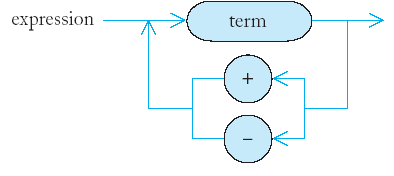• A term is either a factor, or a product or quotient of factors.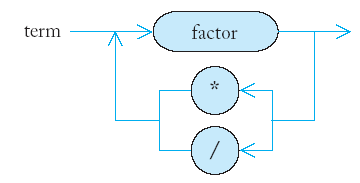• A factor is either a number or an expression closed in parentheses.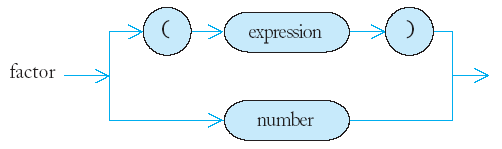## Mutual Recursion

• The syntax diagrams accurately represent which operations should be carried out first.
• First, 4 and 5 should be multiplied, and then the result should be added to 3.
`3 + 4 * 5`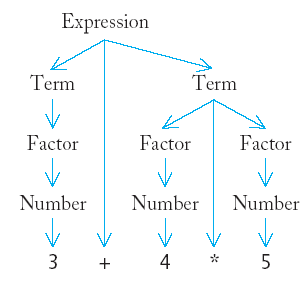• Here, 3 and 4 should be added, and the result should be multiplied by 5.
`(3 + 4) * 5`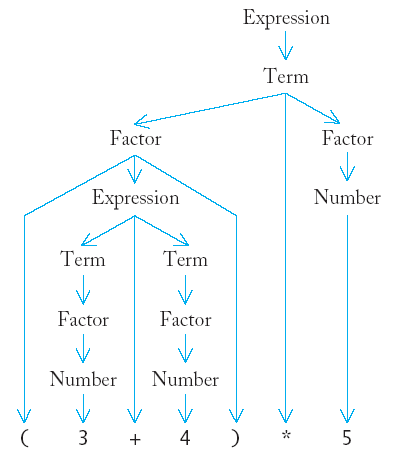## Mutual Recursion

• To compute the value of an expression, we implement three functions expression_value, term_value, and factor_value.
• The expression_value function calls term_value, checks to see if the next input is + or -, and if so calls term_value again to add or subtract the next term.
• The term_value function calls factor_value in the same way, multiplying or dividing the factor values.
• The factor_value function checks whether the next input is '(' or a digit, calling either expression_value recursively or returning the value of the digit.
• The termination of the recursion is ensured because the expression_value function consumes some of the input characters, ensuring that the next time it is called on a shorter expression.
• So, the three functions are mutually recursive.

## The Efficiency of Recursion

• Although recursion can be a powerful tool to implement complex algorithms, it can lead to algorithms that perform poorly.
• We will analyze the question of when recursion is beneficial and when it is inefficient.
• For our study we will examine the Fibonacci sequence of numbers defined by the equations:
• f1 = 1
• f2 = 1
• fn = fn-1 + fn-2
• The first ten terms of the Fibonacci sequence are
1, 1, 2, 3, 5, 8, 13, 21, 34, 55
• One can easily write a recursive function to compute Fibonacci numbers.
`int fib(int n){  if (n <= 2) return 1;   else return fib(n - 1) + fib(n - 2);}`
• The function above works correctly, but for moderately large values of n it takes an amazing long time to compute the value.
• Try running a test program with n between 30 and 50 to see this effect.
• To determine the problem, we insert trace messages into the function.
`int fib(int n){  cout << "Entering fib: n = " << n << "\n";   int f;   if (n <= 2) f = 1;   else f = fib(n - 1) + fib(n - 2);   cout << "Exiting fib: n = " << n      << " return value = " << f << "\n";   return f;}`
• The output from the function indicates why the computation takes so long.
`Entering fib: n = 6Entering fib: n = 5Entering fib: n = 4Entering fib: n = 3Entering fib: n = 2Exiting fib: n = 2 return value = 1Entering fib: n = 1Exiting fib: n = 1 return value = 1Exiting fib: n = 3 return value = 2Entering fib: n = 2Exiting fib: n = 2 return value = 1Exiting fib: n = 4 return value = 3Entering fib: n = 3Entering fib: n = 2Exiting fib: n = 2 return value = 1Entering fib: n = 1Exiting fib: n = 1 return value = 1Exiting fib: n = 3 return value = 2Exiting fib: n = 5 return value = 5Entering fib: n = 4Entering fib: n = 3Entering fib: n = 2Exiting fib: n = 2 return value = 1Entering fib: n = 1Exiting fib: n = 1 return value = 1Exiting fib: n = 3 return value = 2Entering fib: n = 2Exiting fib: n = 2 return value = 1Exiting fib: n = 4 return value = 3Exiting fib: n = 6 return value = 8`
• The call tree helps illustrate how the functions call one another.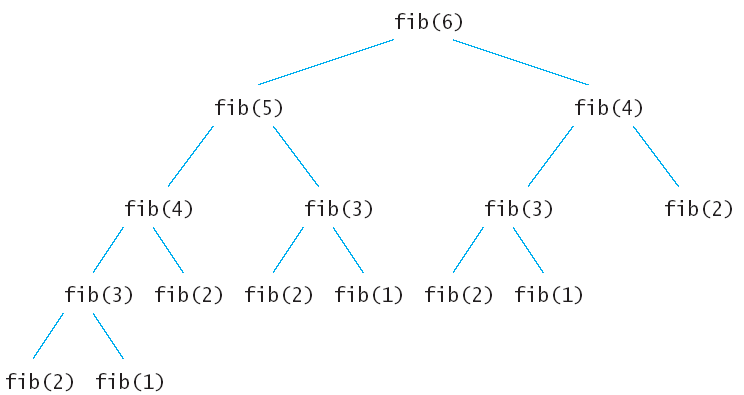• Because the fib(4) is called twice and fib(3) is called three times, the functions spends a lot of time needlessly computing the same values over and over.
• A person would just write down the values as they were computed and add up the last two to get the next one; no sequence value would ever be computed twice.
• To imitate the pencil-and-paper process, you would write a loop to compute Fibonacci numbers.
`int fib(int n){  if (n <= 2) return 1;   int fold = 1;   int fold2 = 1;   int fnew;   for (int i = 3; i <= n; i++)   {  fnew = fold + fold2;      fold2 = fold;      fold = fnew;   }   return fnew;}`
• Can you always speed up a recursive solution by changing it into a loop?
• Frequently, the iterative and recursive solution have essentially the same performance.
• For example, an iterate solution for the palindrome test:
`bool is_palindrome(string s){  int start = 0;   int end = text.length() - 1;   while (start < end)   {  if (s[start] != s[end] return false;      start++;      end--;   }   return true;}`
• The iterative and the recursive solutions to the palindrome problem run at about the same speed.
• There are quite a few problems that are dramatically easier to solve recursively that iteratively.
• Solving the permutation problem iteratively yields code that is complex, but no faster.
• Often, recursive solutions are easier to understand and implement correctly than their iterative counterparts.
• "To iterate is human, to recurse divine." L. Peter Deutsch.